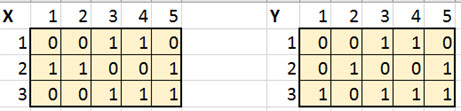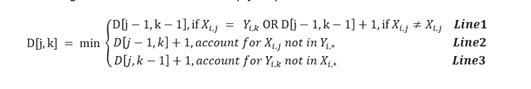# CTU Online Algorithms: Pseudo code

Key Assignment First Draft

Create a portfolio that includes all previous IPs. Add to this portfolio the design of an algorithm that compares one picture with another using dynamic programming.

Your algorithms group has been tasked with creating an app that performs special operations on images. Specifically, your app will compare one black-and-white image into another black-and-white image. There are a number of methods that can be used to perform this task, but your group has agreed that using dynamic programming is a fast and elegant scheme to solve this problem.

Assignment

Design an algorithm (using pseudocode) that takes in as an input, two 2-D int arrays that are assumed to be 2 black-and-white images: initialImage x, whose dimensions are IxJ, and finalImage y, whose dimensions are IxK. The algorithm will compare x to the y, row-by-row, as defined below. Your algorithm will employ a dynamic programming scheme to compare X to Y identifying the minimal difference between each row.

Because you are working with black-and-white images only, you should assume that each image is a 2-D int array consisting of 2 possible values: 0 or 1, where 0 represents black and 1 represents white. Thus, this 2-D grid of 0 and 1 values comprise a 2-D black-and-white image. Each row of this image is then simply a 1-D int array filled with either 0s or 1s. Therefore, you must define how you will measure the difference between the strings of 0s and 1s in each row.

Remember that you will do the comparison one row in the images at a time.

First, compare X1,* to Y1,*. (Here X1,* is the first row in image X and Y1,* is the first row in image Y ). Next, compare X2 to Y2… Each one of these comparisons will require the construction of a D (distance) matrix.

In the following example, the first row of X is X1,*, and the first row of Y is Y1,* = 00110.Use the following recurrence relation to develop your pseudocode:After the D matrix is completed, the minimum number in the bottom row is the minimal mismatch for this row. You will assign this value to the variable minVali. This number tells how different row X1,* is from row Y1,* . You will then repeat this comparison for all rows i and aggregate the difference when complete into variable totalDifference = Si minVali.

As a result, the algorithm will compare the total difference to a threshold value called thresh. If total value is above the threshold, the images are declared different; otherwise, they are declared to be similar images. You can assume that the thresh variable is supplied as an input to your algorithm.

Part 1

Create a portfolio that includes all previous IPs.

Part 2a

Design pseudocode for the image comparison algorithm discussed above, given input Images X, Y, and thresh. The output is a declaration: The images are similar, or The images are different.

Part 2b

Discuss the optimality of the dynamic programming solution. Discuss the time complexity of this algorithm in terms of the size of the inputs X and Y.